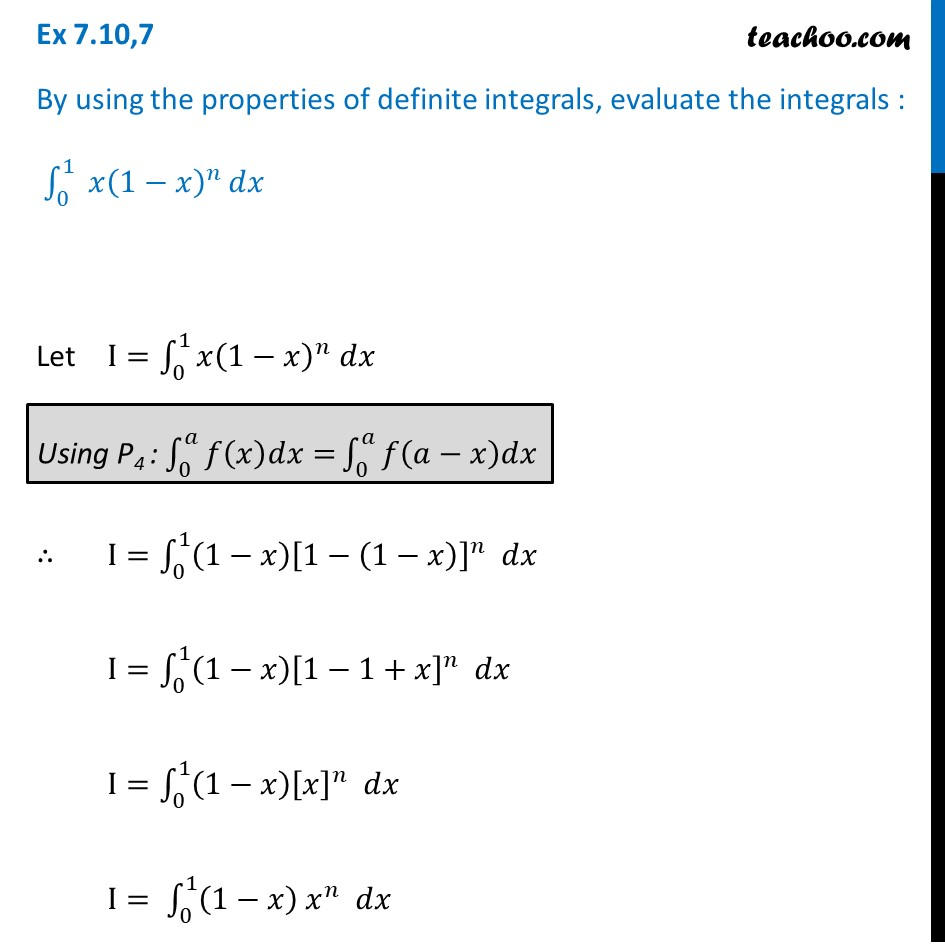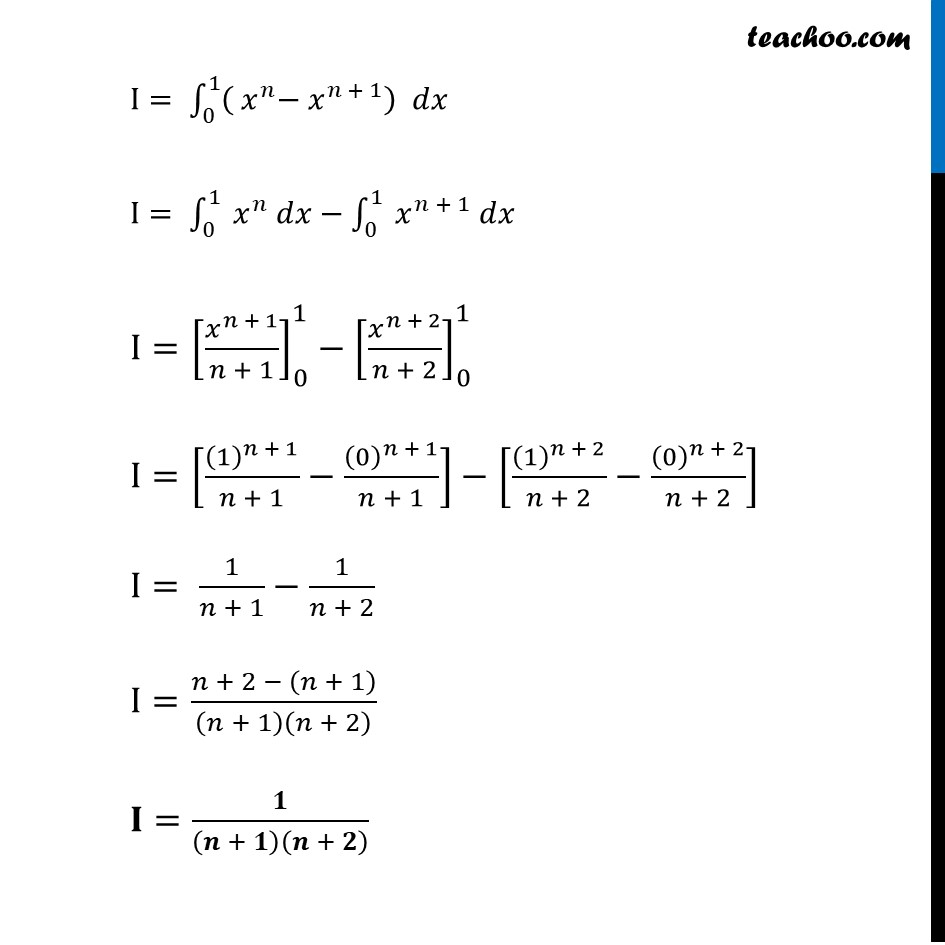Definite Integration by properties - P4

Chapter 7 Class 12 Integrals
Concept wiseLearn in your speed, with individual attention - Teachoo Maths 1-on-1 Class

### Transcript

Ex 7.10,7 By using the properties of definite integrals, evaluate the integrals : ∫_0^1▒〖 𝑥(1−𝑥)^𝑛 〗 𝑑𝑥 Let I=∫_0^1▒〖𝑥(1−𝑥)^𝑛 𝑑𝑥〗 ∴ I=∫_0^1▒〖(1−𝑥) [1−(1−𝑥)]^𝑛 𝑑𝑥〗 I=∫_0^1▒〖(1−𝑥) [1−1+𝑥]^𝑛 𝑑𝑥〗 I=∫_0^1▒〖(1−𝑥) [𝑥]^𝑛 𝑑𝑥〗 I= ∫_0^1▒〖(1−𝑥) 〖 𝑥〗^𝑛 𝑑𝑥〗 I= ∫_0^1▒〖(〖 𝑥〗^𝑛− 𝑥^(𝑛 + 1) ) 𝑑𝑥〗 I= ∫_0^1▒〖〖 𝑥〗^𝑛 𝑑𝑥〗−∫_0^1▒〖〖 𝑥〗^(𝑛 + 1) 𝑑𝑥〗 I=[𝑥^(𝑛 + 1)/(𝑛 + 1)]_0^1−[𝑥^(𝑛 + 2)/(𝑛 + 2)]_0^1 I=[(1)^(𝑛 + 1)/(𝑛 + 1)−(0)^(𝑛 + 1)/(𝑛 + 1)]−[(1)^(𝑛 + 2)/(𝑛 + 2)−(0)^(𝑛 + 2)/(𝑛 + 2)] I= 1/(𝑛 + 1)−1/(𝑛 + 2) I=(𝑛 + 2 − (𝑛 + 1))/(𝑛 + 1)(𝑛 + 2) 𝐈=𝟏/(𝒏 + 𝟏)(𝒏 + 𝟐)